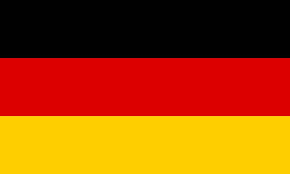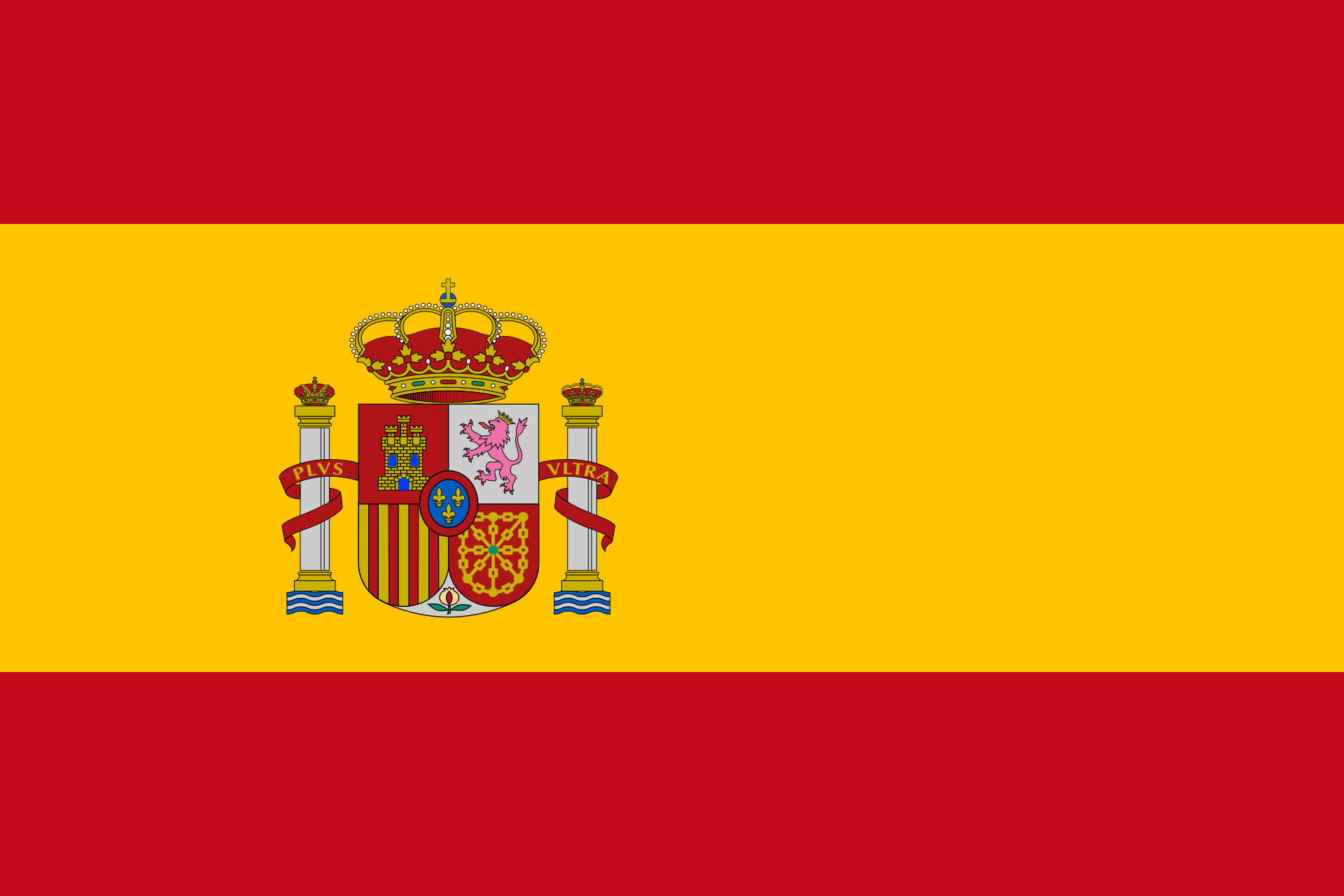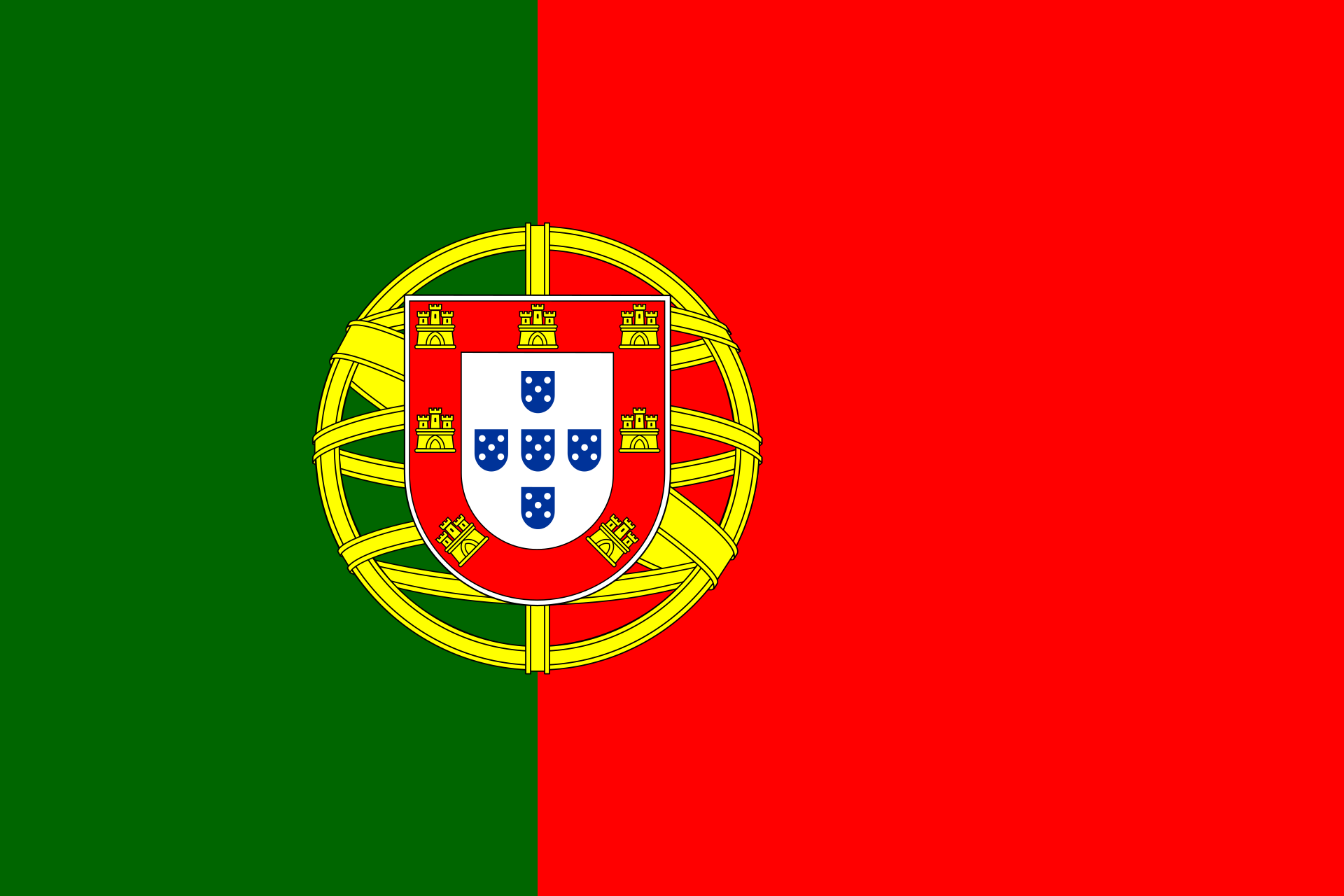# Decimal fractionsThis calculator transforms fractions into decimal numbers and vice versa

Enter a fraction (e.g. 2/3) or a decimal number. Mathepower converts it.

Hints:

If you enter 3/7 , you getIf you enter 1 4/5 , you get
 1 4 5

If you enter 0,_3 , you get   0,3  = 0, 333333....
If you enter 3,42_56 , you get   3.4256

The sign _ says that all further signs are below the overline.

To put a fraction into a decimal number, you just have to divide the numerator by the denominator.

## How to transform a fraction into a decimal number?

It is very simple for fractions that can be expanded to have a 1 followed by only zeroes in the denominator. But this is only possible if the denominator has 2 and 5 as only prime factorsl
Then you do the following:
First expand the fraction so that it has a power of 10 (i.e. a 1 followed by zeroes) in the denominator
Then count how many zeroes the power of 10 has. This is exactly the amount of numbers after the decimal sign.